# Skewb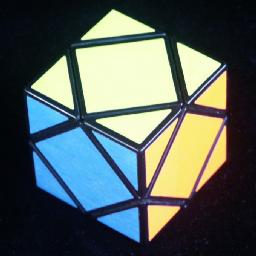The Skewb is a cube where you don't turn the faces, but instead turn exactly half the cube around the corners. The cube is bisected 4 ways, perpendicular to each of the 4 main diagonals. It therefore consists of 8 corner pieces and 6 square face pieces.

This puzzle was invented by Tony Durham. When Uwe Mèffert originally started to produce it, it was called the Pyraminx Cube, but Douglas Hofstadter coined the name Skewb in his column in the Scientific American (July 1982). This column is reprinted in his book MetaMagical Themas.

The Skewb is closely related to the Pyraminx, which also has 4 axes of rotation. The six edge pieces of the pyraminx correspond to the 6 square faces of the Skewb, and the four corner pieces (without the tips) correspond to four of the corners of the Skewb. On the Halpern-Meier pyramid the 4 centres correspond to the other 4 Skewb corners except that rotation of the centres is not visible. Note that the solutions below will only use turns of 4 non-adjacent of the corners of the Skewb, i.e. four which correspond to the corners of the pyraminx.

The Skewb also comes in other forms, like the Creative Puzzleball, Mickey's Challenge, and several other spherical ones. The Skewb Diamond is an octahedral version, and the Ultimate Skewb a dodecahedral one. All these puzzles have essentially the same pieces, which differ only in their colouring. Sometimes there are identical pieces, or the orientation of some pieces are visible on one puzzle but not on another variant. The solutions below are designed for the standard Skewb, in which the orientation of the faces does not matter. If you have a Skewb variant where the face orientation does matter, like some of the puzzleball variants, then the solution to the Ultimate Skewb will be more appropriate.

The Beachball, Diamond and Ultimate Skewb have separate pages devoted to them.

## The number of positions of the standard Skewb:

There are 4 fixed corners with 3 orientations each, 4 free corners with 3 orientations each and 6 face pieces giving a maximum of 6!·4!·38 positions. This limit is not reached because
• The total twist of the corners is fixed (3)
• The faces must have an even permutation (2)
• The free corners must have an even permutation, and hence form a tetrad (2)
• The orientations of the fixed corners and the position of one of the free corners will determine the positions of the other three (3)

Another way of describing the last condition is that the relative position of the two tetrads also defines a kind of twist, which must be equal to the twist of the fixed tetrad. This leaves 6!·4!·36/4 = 3,149,280 positions.

Every position can be solved in at most 11 moves (8.3636 on average). Several years ago I used a computer search to find God's Algorithm, i.e. the shortest solution for each position. Other people have done this also, and the result is shown in the following table:
MovesPositions
01
18
248
3288
41,728
510,248
659,304
7315,198
81,225,483
91,455,856
1081,028
1190
Total      3,149,280

In Sloane's On-Line Encyclopedia of Integer Sequences this is included as sequence A079745.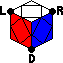## Notation:

Hold the Skewb so that one tetrad of the corners point left, right, down and back. You will only turn these corners, not the others. Note that a move at any of these corners leaves the other three fixed. Denote clockwise moves at the corners by L, R, D and B. Anti-clockwise turns are denoted L', R', D' and B'. Any face can be specified by two letters, e.g. DR is the face between the down and right corners. The other 4 corners can similarly be specified by 3 letters.

There now follow 3 different solutions. The first solution below closely follows that of the pyraminx.

## Solution 1:

 3 top corners 3 3 top faces 12 bottom corner 1 3 bottom faces 6 4 free corners 11 total: 33 moves

Phase 1: Solve 3 top corners

Rotate L, R, and B so that the colours of the corners match.

Phase 2: Solve 3 top faces

1. Find a face piece at the D corner which does not belong there.
2. Hold the puzzle so that the face belongs at the front, the LR position.
3. Rotate D to bring the piece to the front right, in the RD position.
4. Do LD'L' to put it in place.
5. Repeat the above until all three top faces are correct. If necessary, you can use the same sequence to displace incorrectly placed faces.

Phase 3: Solve the D corner.

1. Simply rotate D to make its corner match the others.

Phase 4: Solve the D faces.

1. There are now only three possibilities left for the last 3 faces. They are either solved, or need to be cycled around the D corner in either direction. To move them, do the appropriate sequence:
 Effect Solution 1.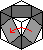Anti-clockwise RLDL'D'R' 2.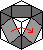Clockwise RDLD'L'R'

Phase 5: Solve the final 4 corners.

1. If the corners are positioned correctly, but only need to be oriented properly, then the following sequences can be used. You will probably need to rotate the whole puzzle to get a position that can be solved by one of the following:
 Effect Solution Alternative solution 1.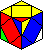DLR-, DRB-, DBL- (L R D')3 (DR'D'R)3 (RL'R'L)3 (LD'L'D)3 2.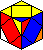DLR+, DRB+, DBL+ (R' L' D)3 (D'LDL')3 (L'RLR')3 (R'DRD')3 3.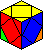DBL-, DRB+ (LDL'D') R (B'D'BD) R' (RL'R'L)3 (LR'L'R)3 4.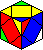DLR+, BLR+, DRB-, DBL- L'B' RD' B'L R'D RB'R' (RD'R'D)3 (BL'B'L)3
2. If the corners are not in the correct position, then one of the following sequences will do:
 Effect Solution Alternative 1 Alternative 2 1.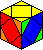DLR->BRL->DLR, LDB->RBD->LDB D' (RL'RL) D (R'BR'B') (LDL'D')3 2.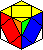DLR->BRL->DLR, LDB->BDR->LDB (LD'L'D) B (L'RLR') B' (L'RLR')3 3.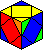DLR->BRL->LRD, LDB->DRB->BLD (D'RDR') (BR'B'R) (RDR'D')3 (DR'D'R)3 4.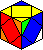DLR->BRL->LRD, LDB->RBD->BLD (LD'L'D) (R'DRD') (DL'D'L)3 (LRL'R')3 5.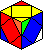DLR->BRL->RDL, LDB->DRB->DBL R' (LD'L'D) L'RL (DR'D'R)3 (RB'R'B)3 6.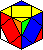DLR->BRL->LRD, LDB->BDR->BLD L (R'DRD') RL'R' (D'LDL')3 (L'BLB')3 7.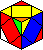DLR->LBR->LRD, LDB->BDR->BLD (L'DLD') R' (DL'D'L) R (B'LBL')3 (LDL'D')3 (BL'B'L)3 8.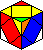DLR->RLB->DLR, LDB->BDR->LDB (D'LDL') B (RL'R'L) B' (DL'D'L)3 (LR'L'R)3 (DL'D'L)3 R (DL'D'L)3 R' 9.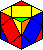DLR->RLB->DLR, LDB->DRB->LDB D' (LR'L'R) D (L'BLB') (R'DRD')3 (L'RLR')3 (R'DRD')3 B (DL'D'L)3 B'

## Solution 2:

 3 top corners 3 3 top faces 12 top corner 6 bottom corner 1 bottom layer 11 total: 33 moves

Phases 1-2:

See solution 1.

Phase 3: Place the top corner correct.

1. Find the piece belonging in the top corner.
2. If it is in place but incorrectly oriented, then do one of the following:
 Effect Solution 1.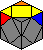BRL- LD'L' R'D'R 2.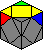BRL+ R'DR LDL'
3. If the top corner piece is not in place then turn D to bring it to the LRD position, and do one of the following:
 Effect Solution 1.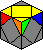DLR->BRL B' D'L'DL B 2.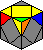DLR->RLB B' L'D'LD B 3.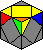DLR->LBR B RDR'D' B'

Phase 4: Solve the D corner.

1. Simply rotate D to make its corner match the others.

Phase 5: Solve the bottom layer.
There are three categories, depending on the position of the faces. For each sequence the effect on the corner twists is given.

1. If the final three faces are correctly positioned, then do one of the following:
 Effect Solution 1.DBL-, DRB+ (LDL'D') R (B'D'BD) R' 2.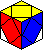DBL+, DRB- R (D'B'DB) R' (DLD'L') 3.DBL+, DRB+, DLR+ (R' L' D)3 4.DBL-, DRB-, DLR- (L R D')3
2. If the final 3 faces need a clockwise twist around D, then do one of the following:
 Effect Solution 1.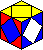Corners are correct RL' BDB'D' RLR 2.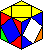DBL-, DRB+ (R'D'RD) (RL'R'L) 3.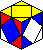DBL+, DRB- (RL'R'L) (DLD'L') 4.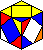DBL+, DRB+, DLR+ B'LBL RD' RL'RL' D 5.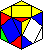DBL-, DRB-, DLR- R'LR' B'L' RD'RD B
3. If the final 3 faces need an anti-clockwise twist around D, then do one of the following:
 Effect Solution 1.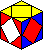Corners are correct L'R B'D'BD L'RL' 2.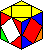DBL-, DRB+ (LDL'D') (L'RLR') 3.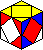DBL+, DRB- (L'RLR') (D'R'DR) 4.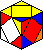DBL+, DRB+, DLR+ B' D'R'DR' LBRL'R 5.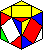DBL-, DRB-, DLR- BR'B'R' L'DL'RL'RD'

## Solution 3:

 3 top corners 3 top corner 5 bottom corner 1 3 bottom corners 6 6 faces 18 total: 32 moves

Phase 1:

See solution 1.

Phase 2: Solve the top corner

1. Find the piece belonging in the top corner.
2. If it is in place but incorrectly oriented, then do one of the following:
 Effect Solution 1.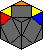BRL- RDRDR 2.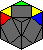BRL+ R'D'R'D'R'
3. If the top corner piece is not in place then turn D to bring it to the LRD position, and do one of the following:
 Effect Solution 1.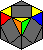DLR->BRL D'RD'R' 2.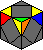DLR->RLB R'D'R 3.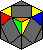DLR->LBR LDL'

Phase 3: Solve the D corner.

1. Simply rotate D to make its corner match the others.

Phase 4: Orient the bottom corners.

1. If two corners need to be twisted, then hold the puzzle so that they are at the DBL and DBR positions. Do one of the following:
 Effect Solution 1.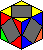DBL-, DRB+ LR'L' D'RD 2.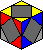DBL+, DRB- D'R'D LRL'
2. If all three corners need to be twisted then do one of the following:
 Effect Solution 1.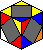DBL+, DRB+, DLR+ R'L'R'L'R'L' 2.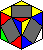DBL-, DRB-, DLR- LRLRLR

Phase 5: Position the faces

1. Here is a list of sequences that move the faces about. They either swap two pairs of faces, of cycle three faces. You can solve any face position by applying at most two of these.
 Effect Solution 1.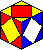DL-LR DR-DB D'R'DR DLD'L' 2.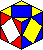DL-DB DR-LR R'D'RDLDL'D' 3.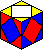DL-DR DB-LR D' R'D'RD LDL' 4.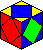DR->BL->LR->DR R'D'RD B'L'BL 5.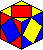DL->DR->LR->DL R' D'L'DL B'R'BR'